Search for on the web shopping
Tue, 31 Jan, 2023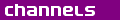Top links - Sudoku - Collectibles - PSP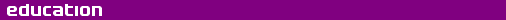Electron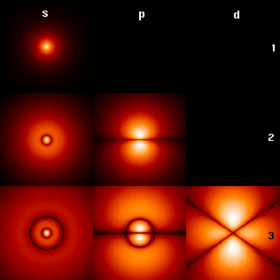The first few hydrogen atom electron orbitals shown as cross-sections with colour-coded probability density
Classification
 Elementary particle Fermion Lepton First Generation Electron
Properties
 Mass: 9.109 3826(16) × 10−31 kg 1⁄1836.152 672 61(85) amu 0.510 998 918(44) MeV/ c2 Electric Charge: −1.602 176 53(14) × 10−19 C Spin: ½ Color Charge: none Interaction: Gravity, Electromagnetic, Weak
The electron is a fundamental subatomic particle that carries a negative electric charge.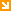Jump to Page Contents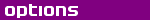Pay as you go No monthly charges. Access for the price of a phone call Go> Unmetered Flat rate dialup access from only £4.99 a month Go> Broadband Surf faster from just £13.99 a month Go>

 This weeks hot offer24: Series 5 In association with Amazon.co.uk £26.97

ContentsOverviewIn practiceIn the laboratoryIn theoryHistoryOverview - Contents

Within an atom, the electrons surround the nucleus of protons and neutrons in a circular pattern known as an electron shell. The word electron was coined in 1894 and is derived from the term electric force introduced by William Gilbert. Its origin is the Greek word 'ηλεκτρον, meaning amber.Electrons in motion constitute electric current, which may be used by scientists and engineers to measure many physical properties. Electric current existing for a finite time gives rise to a movement of charge (electricity) that may be harnessed as a practical means to perform work.The understanding of electrons has changed dramatically over the centuries, the most significant perhaps being the development of quantum mechanics in the 20th century and the idea of particle wave duality, that is, electrons can exhibit wave-like and particle like properties. Equally as important, particle physics has also furthered understanding of the electron immeasureably.The variations in electric field generated by differing numbers of electrons and their configurations in atoms determine the chemical properties of the elements. These fields play a fundamental role in chemical bonds and chemistry.In practice - Contents

Classification
The electron is one of a class of subatomic particles called leptons, which are believed to be fundamental particles (that is, they cannot be broken down into smaller constituent parts). The word "particle" is somewhat misleading, however, because quantum mechanics shows that electrons also behave like a wave, e.g., in the double-slit experiment; this is called wave-particle duality.The antiparticle of an electron is the positron, which has the same mass but positive rather than negative charge. The term negatron is sometimes used to refer to standard electrons so that the term electron may be used to describe both positrons and negatrons, as proposed by Carl D. Anderson. Under ordinary circumstances, however, electron refers to the negatively charged particle alone.

Properties and behaviour
Electrons have a negative electric charge of −1.6 × 10−19 coulombs, and a mass of about 9.11 × 10−31 kg (0.51 MeV/c2), which is approximately 1/1836 of the mass of the proton. These are commonly represented as e.According to quantum mechanics, electrons can be represented by wavefunctions, from which the electron density can be determined. Each electron has its own wavefunction, which is called an orbital. The exact momentum and position of an electron cannot be simultaneously determined. This is a limitation described by the Heisenberg uncertainty principle, which, in this instance, simply states that the more accurately we know a particle's position, the less accurately we can know its momentum, and vice versa.The electron has spin ½ and is a fermion (it obeys Fermi-Dirac statistics). In addition to its intrinsic angular momentum, an electron has a magnetic moment along its spin axis.While most electrons are found in atoms, others move independently in matter, or together as an electron beam in a vacuum. In some superconductors, electrons move in Cooper pairs, in which their motion is coupled to nearby matter via lattice vibrations called phonons. When electrons move, free of the nuclei of atoms, and there is a net flow, this flow is called electricity, or an electric current.A body has a static charge when the body has more or fewer electrons than are required to balance the positive charge of the nuclei. When there is an excess of electrons, the object is said to be negatively-charged. When there are fewer electrons than protons, the object is said to be positively-charged. When the number of electrons and the number of protons are equal, their charges cancel each other and the object is said to be electrically neutral. A macroscopic body can acquire charge through rubbing, i.e., the phenomena of triboelectricity. Electrons and positrons can annihilate each other and produce a pair of photons. However, high-energy photons may transform into an electron and a positron by a process called pair production.The electron is an elementary particle — it has no substructure (at least, experiments have not found any so far, and there is good reason to believe that there is not any). Hence, it is usually described as point-like, i.e., with no spatial extension. However, if one gets very near an electron, one notices that its properties ( charge and mass) seem to change. This is an effect common to all elementary particles: The particle influences the vacuum fluctuations in its vicinity, so that the properties one observes from far away are the sum of the bare properties and the vacuum effects (see renormalization).There is a physical constant called the classical electron radius, with a value of 2.8179 × 10−15 m. Note that this is the radius that one could infer from its charge if the physics were only described by the classical theory of electrodynamics and there were no quantum mechanics (hence, it is an outdated concept that nevertheless sometimes still proves useful in calculations).The speed of an electron in a vacuum can approach, but never reach c, the speed of light in a vacuum. This is due to an effect of special relativity. The effects of special relativity are based on a quantity known as gamma or the Lorentz factor. Gamma is a function of v, the velocity of the particle, and c. The following is the formula for gamma:$gamma = 1 / sqrt{1 - (v^2/c^2)}$
The energy necessary to accelerate a particle is gamma minus one times the rest mass. For example, the linear accelerator at Stanford can accelerate an electron to roughly 51 GeV. This gives you a gamma of 100,000 given that the rest mass of an electron is 0.51 MeV/c² (the relativistic mass of this fast electron is 100 000 times its rest mass). Solving the equation above for the speed of the electron gives a speed of:$left(1-frac {1} {2} gamma ^{-2}right)c$ = 0.999 999 999 95 c.
(The formula applies for large γ.)

In the universe
It is believed that the number of electrons existing in the known universe is at least 1079. This number amounts to a density of about one electron per cubic metre of space.Based on the classical electron radius and assuming a dense sphere packing, it can be calculated that the number of electrons that would fit in the observable universe is on the order of 10130. Of course, this number is even less meaningful than the classical electron radius itself.

In industry
Electron beams are used in welding as well as lithography.In the laboratory - Contents

Early experiments
The quantum or discrete nature of electron's charge was observed by Robert Millikan in the Oil-drop experiment of 1909.

Usage
Electron microscopes are used to magnify details up to 500,000 times. Quantum effects of electrons are used in Scanning tunneling microscope to study features at the atomic scale.In theory - Contents

In relativistic quantum mechanics, the electron is described by the Dirac Equation. Quantum electrodynamics (QED) models an electron as a charged particle surrounded by a sea of interacting virtual particles, modifying the sea of virtual particles which makes up a vacuum. Although this theory involves difficult theoretical problems where calculations produce infinite terms, a practical (although mathematically dubious) method called renormalization was discovered whereby infinite terms can be cancelled to produce finite predictions about the electron. The correction of just over 0.1% to the predicted value of the electron's gyromagnetic ratio from exactly 2 (as predicted by Dirac's single particle model), and its extraordinarily precise agreement with the experimentally determined value, is viewed as one of the pinnacles of modern physics. There are now indications that string theory and its descendants may provide a model of the electron and other fundamental particles where the infinities in calculations do not appear, because the electron is no longer seen as a dimensionless point. At present, string theory is very much a 'work in progress' and lacks predictions analogous to those made by QED that can be experimentally verified.In the Standard Model of particle physics, it forms a doublet in SU(2) with the electron neutrino, as they interact through the weak interaction. The electron has two more massive partners, with the same charge but different masses: the muon and the tau lepton.The antimatter counterpart of the electron is its antiparticle, the positron. The positron has the same amount of electrical charge as the electron, except that the charge is positive. It has the same mass and spin as the electron. When an electron and a positron meet, they may annihilate each other, giving rise to two gamma-ray photons, each having an energy of 0.511 MeV (511 keV). See also Electron-positron annihilation.Electrons are also a key element in electromagnetism, an approximate theory that is adequate for macroscopic systems, and for classical modelling of microscopic systems.History - Contents

The electron as a unit of charge in electrochemistry was posited by G. Johnstone Stoney in 1874, who also coined usage of "electron" in 1894.The discovery that the electron was a subatomic particle was made in 1897 by J.J. Thomson at the Cavendish Laboratory at Cambridge University, while he was studying " cathode rays". Influenced by the work of James Clerk Maxwell, and the discovery of the X-ray, he deduced that cathode rays existed and were negatively charged "particles", which he called "corpuscles." He published his discovery in 1897.The periodic law states that the chemical properties of elements largely repeat themselves periodically and is the foundation of the periodic table of elements. The law itself was initially explained by the atomic mass of the elements. However, as there were anomalies in the periodic table, efforts were made to find a better explanation for it. In 1913, Henry Moseley introduced the concept of the atomic number and explained the periodic law with the number of protons each element has. In the same year, Niels Bohr showed that electrons are the actual foundation of the table. In 1916, Gilbert Newton Lewis explained the chemical bonding of elements by electronic interactions.
Change Text Size:
[A] [default] [A]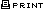| |